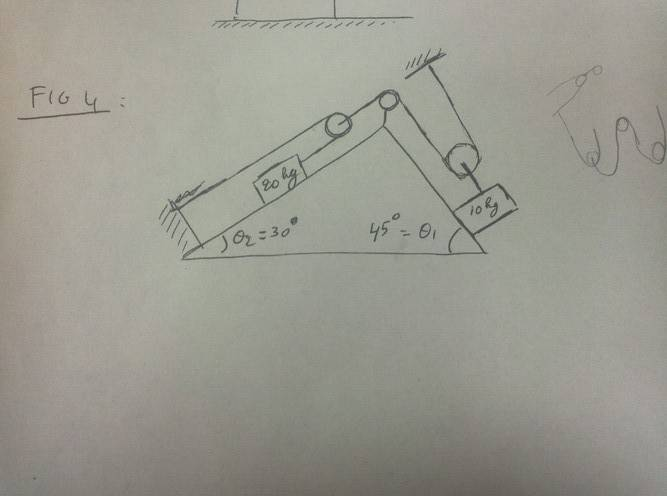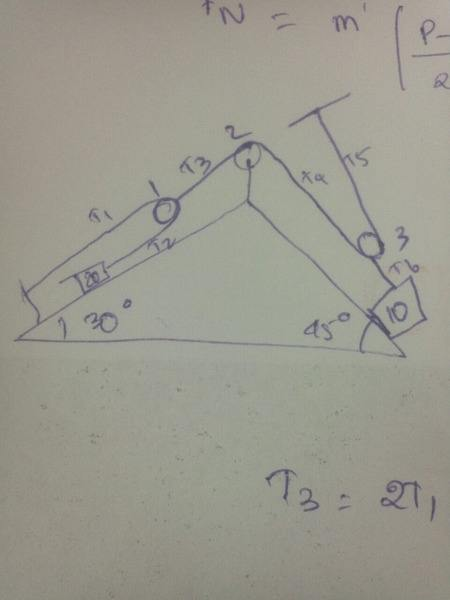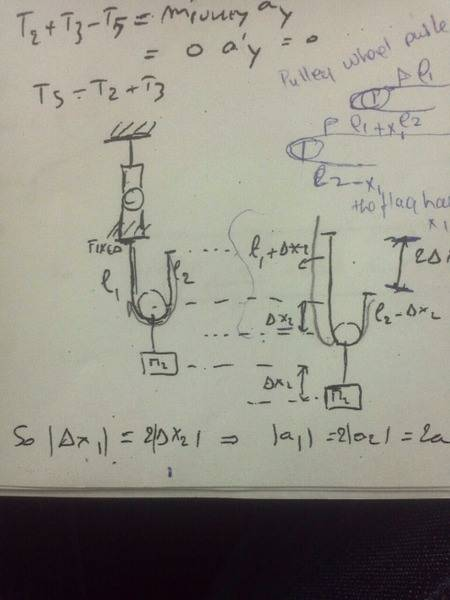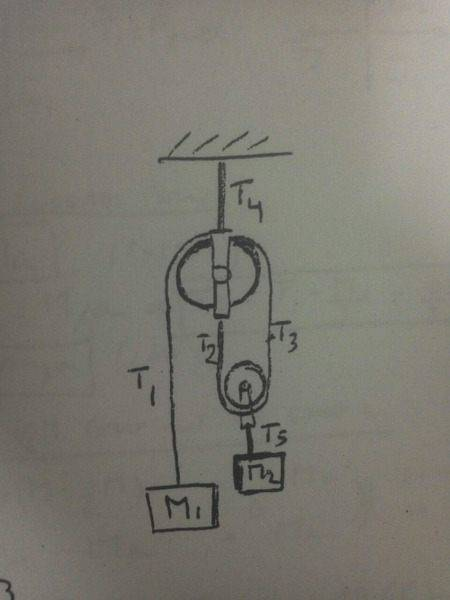# Blocks, Pulley, Ropes, and Tension

## Homework Statement

Look at figure 4Two blocks are attached to mass-less ropes and pulleys that have negligible mass and negligible friction.
a. Find the connection between the tensions in the ropes.
b. Find the connection between the accelerations of the blocks.
c. Calculate acceleration of each block.
d. Calculate tension in each rope.

F= ma

## The Attempt at a Solution

I did FBD for pulleys and the masses.
For part a I got,So here I said that T3=T4=T5.
T3=2(T2) (because T3= 2(T1) and T1=T2)
2(T3)=T6

For part b,
The FBD for m= 20 kg gave me,
T2-W1sin30= m1a1 in the x-direction

and the FBD for m=10 gave me
W2sin45-T6=m2a2 in the x-direction.

My teacher told me something about finding the connection between the accelerations of the masses. He said that the if a pulley accelerates over a certain distance x then the other moves over the distance L-x where L is length of the rope. Something alone these lines and for one problem we did in class he found connection between accelerations like accofblock1=2accofblock2. Something like that. I wanted to know this. I know that if I know how to find this, I can go about the rest of the two parts pretty well. Any idea?

## Answers and Replies

The basic idea is this: you move one block by a distance$x$ in your favorite direction, you calculate how the other block _must_ move in order to keep the ropes taut, say it moves $y$. Once you have a relation between $x$ and $y$, you can relate their acceleration quite easily.

Please explain a little more further. It's still not clear to me.

If I move the block with m=10 kg a distance of x meters DOWNWARD, then the 20 kg will move x meters upwards as well, is that what you're trying to say here? So acceleration of 10 kg= twice the acceleration of 20 kg? I mean how does the acceleration relate. I'm confused.

Also can you check and tell me if the I've done part a correctly?

The only way I see the motion is that if the 10 kg block moves, then the 20 kg block moves as well. So let's say the 10 kg block moves a distance x meters, then the pulley at the top, pulls the pulley on the left side that moves the 20 kg block upwards. This is how I see it. So if the 10 kg block moves x meters, then the 20 kg block also moves x meters. But since its more heavier, does it mean that it moves slower/lesser? How? Does the number of pulleys have to do something with the acceleration?

Please explain a little more further. It's still not clear to me.

If I move the block with m=10 kg a distance of x meters DOWNWARD, then the 20 kg will move x meters upwards as well, is that what you're trying to say here?
You are correct till here.
So acceleration of 10 kg= twice the acceleration of 20 kg? I mean how does the acceleration relate. I'm confused.

Also can you check and tell me if the I've done part a correctly?

If $x_{10}$ = -$x_{20}$, how do the accelerations relate? (Remember, $a$ = $\frac{d^2x}{dt^2}$)

Still doesn't ring a bell. I mean, if 10kg block is moving down by x then shouldn' that be -ve. And 20 kg is move upwards by x and that has to be positive. Either way the equation looks fine. Until the part where you brought up the derivative. Acceleration is change in velocity over time and velocity is change in distance over time. There's no mention of time here. So lets say they move a distance of x over Δt. So, 10 kg moves by x meters and 20 kg by -x meters (as you said here... Still not sure why). So we will have, x-(-x)/Δt. Which is 2x.

Is that the acceleration? Where does the Δt go? So, acceleration of 10 kg block is twice of 20 kg block? Still confused.Last edited:
You are correct till here.

If $x_{10}$ = -$x_{20}$, how do the accelerations relate? (Remember, $a$ = $\frac{d^2x}{dt^2}$)

Still doesn't ring a bell. I mean, if 10kg block is moving down by x then shouldn' that be -ve. And 20 kg is move upwards by x and that has to be positive. Either way the equation looks fine. Until the part where you brought up the derivative. Acceleration is change in velocity over time and velocity is change in distance over time. There's no mention of time here. So lets say they move a distance of x over Δt. So, 10 kg moves by x meters and 20 kg by -x meters (as you said here... Still not sure why). So we will have, x-(-x)/Δt. Which is 2x.

Is that the acceleration? Where does the Δt go? So, acceleration of 10 kg block is twice of 20 kg block? Still confused.It doesn't matter what sign of $x$ I choose, but okay, I will rewrite my equation as -$x_{10}$ = $x_{20}$. Does that work?
$t$ the time goes the same for both the blocks (nothing is going at relativistic speeds here). Therefore, -$a_{10}$ = -$\frac{d^2x_{10}}{dt^2}$ = $\frac{d^2x_{20}}{dt^2}$ = $a_{20}$.

So the acc of 20 KG BLOCK= - acc of 10 kg block?
Is that right?

And is the connection between the tensions right?The reason why I am asking is because of this explanation my professor gave me. He showed the connection between the accelerations of the two blocks like this and I didn't understand how.

This is the actual figure to the explanation above. It's different from this problem I posted here.He has shown that the movement from one is X and the other one moves 2X. I am not sure how. and if I apply the same concept to what you're saying then I am getting confused,.

Your confusion is justified. I was not doing the calculation correctly.

But the idea is right. Your professor has explained it mathematically already, my earlier post (below) explained it in English. I will try to explain it more clearly later tonight (unless you figure out that 4$a_{10}$ = $a_{20}$).

The basic idea is this: you move one block by a distance$x$ in your favorite direction, you calculate how the other block _must_ move in order to keep the ropes taut, say it moves $y$. Once you have a relation between $x$ and $y$, you can relate their acceleration quite easily.

Okay sure. Please let me know. I'll be waiting for your reply. I'll try my best to understand it myself as well.